## 3D-Dithering

Ing. Petr Chlumsky
e-mail: chlumsky@cslab.felk.cvut.cz

Department of Computer Science,
Czech Technical University,
Karlovo namesti; 13, 121

### Abstract

3D-Dithering is a special lossy method for voxel model compression. It is highly recommended, for medicinal data, because 3D-Dithering has very low level of degradation, and we can successfully apply another method to Undithered data.

#### Keywords

Data compression, dithering, medicine data

## 1. Introduction

As grow a technical level of computer technology, we can notice problem of processing and archiving very huge volume of electronic data. This problem has been solved by many scientists.

Now we can solve problem of very large volume of information by many of common (Huffman coding, LZW, LZArit etc.  ) or specialised (compression of images or some network compression methods) compression algorithms.

These trends we can see also in computer graphics, e. g. in processing of large voxel models. This work is alternative approach to the problem of voxel model compression.

## 2. Dithering as a Compression Method

Originally, Dithering has been developed for colour reduction in two dimensional pictures.

Dithering as a compression method is a problem, which we can use as for two dimensional pictures as for three dimensional voxel models. In the first step of explanation, I describe how we can use dithering for image compression .

### 2.1 Basic Principles of 2D-Dithering Compression Method

Basic Principles of 2D-Dithering Compression Method we can summarise in four steps.

• In first phase, we decrease volume of information of every pixel element ( to one half, one quarter etc.). This process I will name 2D-Dithering.

• After 2D-Dithering it is possible to compress dithered voxel model with lossless compression method (like Huffman coding, LZW, LZArit, LZHuff etc.  )

• First step of decompression is decoding compressed data with used lossless compression method.

• Last step is reconstruction of dithered picture. This process I will name 2D-UnDithering.

#### 2.1.1 2D-Dithering

For a 2D-Dithering I used Floyd Steinberg Dithering, but it is possible to make some experiments with Ordered Dithering or similar method.

Floyd-Steinberg Dithering and 3D-Dithering have one important feature: precious error distribution.

If original voxel intensity is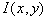and dithered voxel intensity of the same voxel is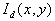, we can defined, that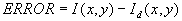.

On the image is the one possibility of distribution of this error information.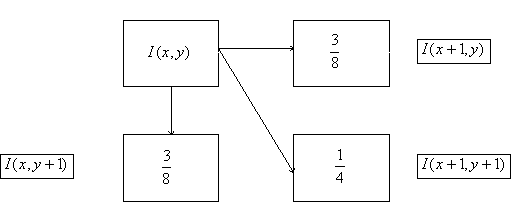Error diffusion in Floyd-Steinberg Dithering

This possibility of error diffusion we can describe via this equations: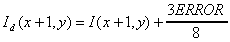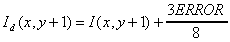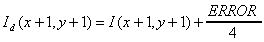#### 2.1.2 2D-UnDithering

2D-Undithering is inverse process for Dithering. This Process we can implement using convolution , .

A

is convolutory matrix with size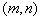. But almost m and n are odd integers. Undithered intensity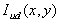we can describe from dithered intensity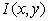as: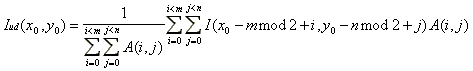Matrix A is an normal convolutory matrix. With good result I used these matrixes: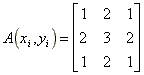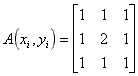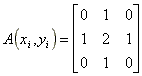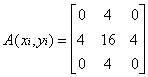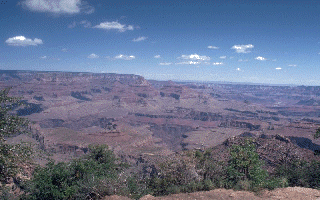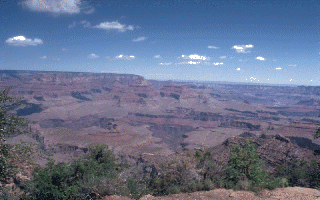On the left side is original picture and on the right side are same picture after decompression using 2D-UnDithering.

### 2.2 Basic Principles of 3D-Dithering Compression Method

Basic Principles of 3D-Dithering Compression Method are very similar with two dimensional dithering, because it is only extension from 2D to 3D.

• In first phase, we decrease volume of information of every volume element ( to one half, one quarter etc.). This process I will name 3D-Dithering.
• After 3D-Dithering it is possible to compress dithered voxel model with lossless compression method (like Huffman coding, LZW, LZArit, LZHuff etc.  )
• First step of decompression is decoding compressed data with used lossless compression method.
• Last step is reconstruction of dithered picture. This process I will name 3D-UnDithering.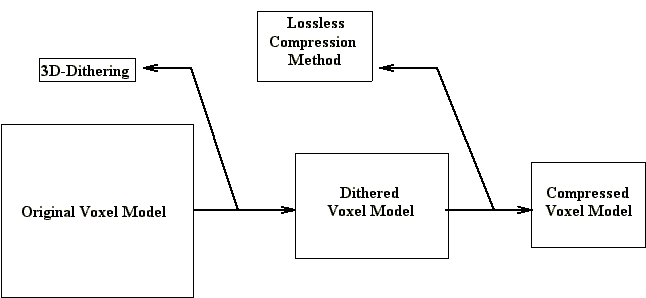Compression method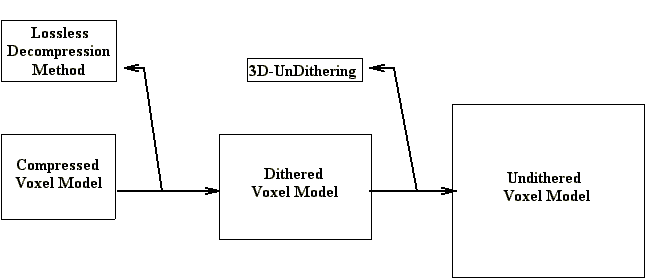Decompression Method

#### 2.2.1 3D-Dithering

For 3D-Dithering is using an algorithm, which is very similar with Floyd-Steinberg Dithering. We can say, that 3D-Dithering is modified Floyd-Steinberg Dithering.

Floyd-Steinberg Dithering and 3D-Dithering have same feature: precious error distribution.

If original voxel intenzity is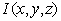and dithered voxel intensity of the same voxel is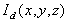, we can defined, that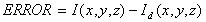. On the image is the one possibility of distribution of this error information.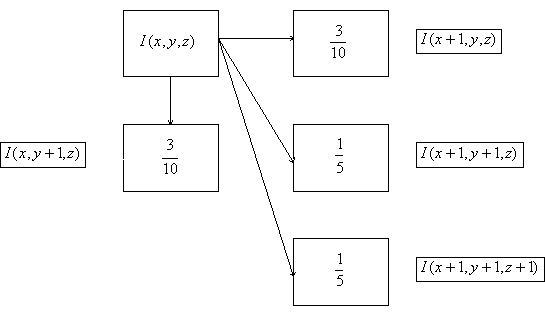Error diffusion in 3D-Dithering

This possibility of error difusion we can describe via this equations: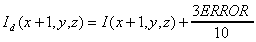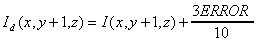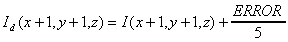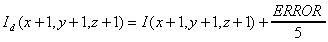The most important thing of this method is, that error is distributed via diagonal and theoretically an error from first processed voxel change an intensity of last processed voxel. This feature minimalized a level of degradation an information at reduction intensity.

#### 2.2.2 3D-UnDithering

For good reconstruction of dithered information we can use one of the methods described below.

##### 2.2.2.1 3D-UnDithering, method one

This method is not fully three dimensional, because it works only with two dimensional convolutory matrixes.

A

is convolution matrix with size. But almost m and n are odd integers. Undithered intensity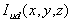we can describe from dithered intensityas: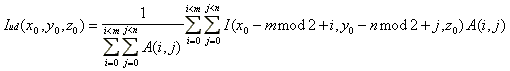Matrix A is an normal convolution matrix. With good result I used this convolution matrixes: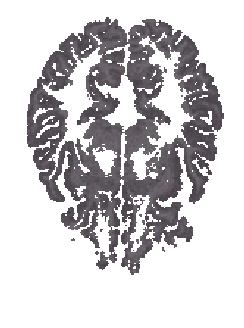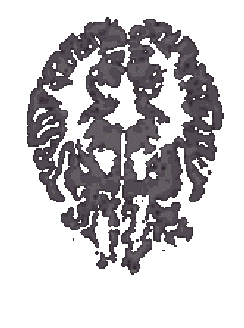On the left side are original data and on the right side are same data after 3D-UnDithering, method one.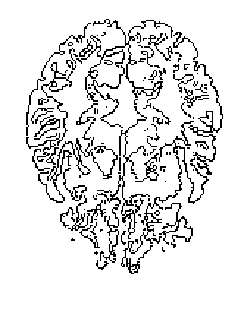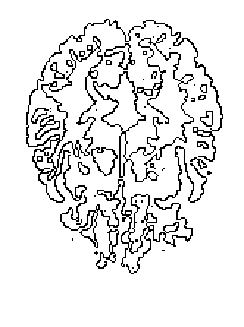On the left side are edges in original data and on the right side are edges in same data after 3D-UnDithering, method one.

3D-UnDithering, method one is very poor method for making undithering in three dimensional space.

• 3D-UnDithering, method one is not adaptable, because this method uses only one convolution matrix for all voxel model. This attribute makes tragically antialiasing.
• Dithering method is fully 3D and undithering method is only 2D.
##### 2.2.2.2 3D-UnDithering, method two

A

is matrix with size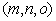. But almost m and n are odd integers and o is integer. Undithered intensitywe can describe from dithered intensityas: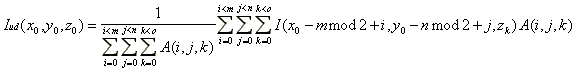As convolution matrixes, we can use these matrixes: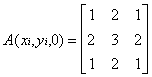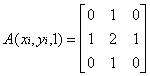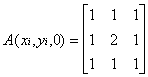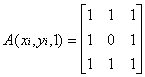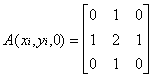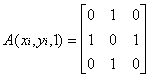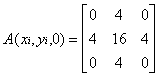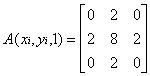3D-UnDithering, method two is better than 3D-Undithering, method one. But this method is not adaptable, because this method uses only one convolutory matrix for all voxel model.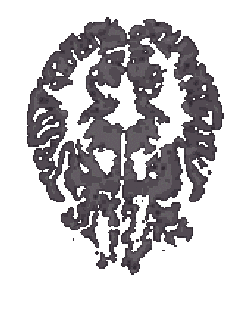On the left side are original data and on the right side are same data after 3D-UnDithering, method two.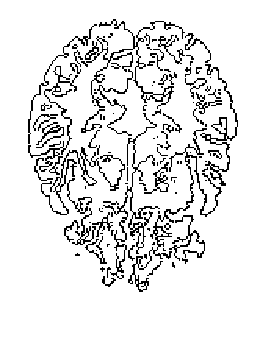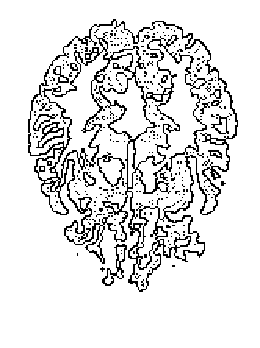On the left side are edges in original data and on the right side are edges in same data after 2D-UnDithering, method two.

## 3. Feature work

3D-Dithering, method one and two are not adaptable, because they use same convolutory matrix for all voxel model. This character makes high level of degradation.

For better quality we can use 3D-UnDithering with dynamic convolutory matrix. The most important thing of this method is, that we must compute origin convolutory matrix for each voxel.

This method will have these features:

• Three dimensional convolutory matrix is computed separately for each voxel. This feature makes this method very adaptable for everyone voxel model.
• Members of convolutory matrix are computed with care to save all edges in voxel model. This feature makes final voxel model very similar to original voxel model.
• We can use rewrite this method for two dimensional images with all features described above.

## 4. Conclusion

This method described has several aspect. It uses two compression steps: 3D-Dithering and lossless compression method. This approach has some features: after first decompression step, we can display voxel model (in low quality). This feature can make faster interactions via network connections.

## 5. References

 Melichar, B., Pokorny, J.: Data Compression, Report DC-92-04,Czech Technical University Prague, Department of Computer Science, 1992

 Stenger, A.:Converting Dithered Image Back to Gray Scale, Dr. Dobb's Journal, Nov. 1992, pp.64-68

 Slavik, P., Prikryl, J.: Dithering as a method for image data compression